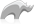##### [ integer ]

Method

The [integer] method returns an integer, the value of which depends on the parameter(s) passed to the method. It has the following variants:

• integer()
• Returns zero
• integer(s::string, base::integer)
• Converts the string parameter into an integer using the base indicator. For example, octal would be 8 while hexadecimal would be 16. One would not include any prefixes on the string value. For example a '0x' or a leading zero for octal.
• integer(d::decimal)
• Decimal is rounded to the nearest integer.
• integer(i::integer)
• Returns the parameter unchanged.
• integer(s::string)
• Converts the string into an integer, respecting any leading - or + operators.
• integer(b::boolean)
• Returns zero or one depending on the parameter value.
• integer(b::bytes)
• Converts the bytes into a string using UTF-8 and then performs as the integer(::string) variant does.
• Syntax
• Methods
• Traits
``````Integer(Value)

local(result = integer(Value))``````
###### Has methods:
• `integer->oncompare(p0::integer)`
• `integer->hash()`
• `integer->+(p0::integer)`
• `integer->+(s::string)`
• `integer->+()::integer`
• `integer->-(p0::integer)`
• `integer->-()`
• `integer->*(p0::integer)`
• `integer->/(p0::integer)`
• `integer->hosttonet16()`
• `integer->hosttonet32()`
• `integer->nettohost16()`
• `integer->nettohost32()`
• `integer->bitset(p0::integer)`
• `integer->bittest(p0::integer)`
• `integer->bitflip(p0::integer)`
• `integer->bitclear(p0::integer)`
• `integer->bitor(p0::integer)`
• `integer->bitand(p0::integer)`
• `integer->bitxor(p0::integer)`
• `integer->bitnot()`
• `integer->bitshiftleft(p0::integer)`
• `integer->bitshiftright(p0::integer)`
• `integer->bytes()`
• `integer->abs()`
• `integer->div(p0::integer)`
• `integer->asString(p0::string, p1::string, p2::string)`
• `integer->asString(-hexaDecimal::boolean = ?, -padding::integer = ?, -padChar::string = ?, -padRight::boolean = ?, -groupchar::string = ?)`
• `integer->dereferencepointer(p0::integer)`
• `integer->asDecimal()`
###### Has traits:
• `any`
• `trait_asString`
• `trait_scalar`
• `trait_serializable`
• Beginner

### To cast an action parameter to type integer:

Values retrieved from an HTML form or URL using [Action_Param] are always of data type string. It is necessary to cast these values to integer if they will be used in a mathematical expression using symbols.

The following example adds the values for two [Action_Param] methods using the math symbol +.

Code

``integer(action_param('Price')) + integer(action_param('Shipping'))``Please note that periodically LassoSoft will go through the notes and may incorporate information from them into the documentation. Any submission here gives LassoSoft a non-exclusive license and will be made available in various formats to the Lasso community.